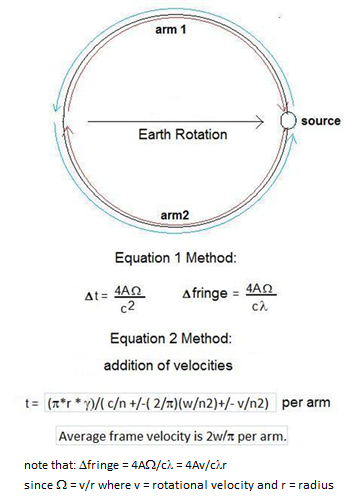Please fill or choose the data marked red and try submitting again.
 Sagnac Interferometer / Fibre-Optic Gyroscope (FOG): Sagnac loop Length (m) Refractive Index of fibreLoop radius (m) wavelength of light number of loops tangential v due to earth: frame Velocity w (m/s) z-axis = vertical m/s Rotational velocity v (m/s) z-axis = NS m/s area of loop (m2): z-axis = EW 0 m/s Angle Wind: Arm 1 forward Time Arm 2 return Time Time round trip: Time Difference Blue -Red 0 0 Fringe Difference: Arm 1 forward Time Arm 2 return Time Time round trip: Arm 1 forward Time Arm 1 forward Time Time round trip: Time Difference Blue-Red 90 Average frame velocity is 2V/p per arm. 90 Fringe Difference Frame velocity for g is 2V/p per arm Arm 1 forward Time Arm 2 return Time Time round trip: General Equation per arm: (p*r * g)/( c/n +/-( 2/p)(w/n2)+/- v/n2) Earth Rotational Velocity: Lorentz Contraction g : Latitude (degrees): min. 0= YES, 1 = No DFringe due to g : m/s ................Earth Rotation Values: ................ ......Regular Rotation/Translation Values:...... Using Eq. 1 Method: Using Eq. 1 Method: Using Eq. 2 Method: Using Eq. 2 Method: DFringe due to Earth Rot. : Dt due to Earth rot. : z-axis = vertical z-axis = vertical z-axis = vertical 4WAsin(lat)/c2 DFringe due to frame vel. w: Dt due to frame velocity w: z-axis = NS 4WAcos(lat)/c2 z-axis = vertical z-axis = vertical DFringe due to rot. vel. v: Dt due to rot. velocity v: z-axis = EW no W in this plane 0.00e+000